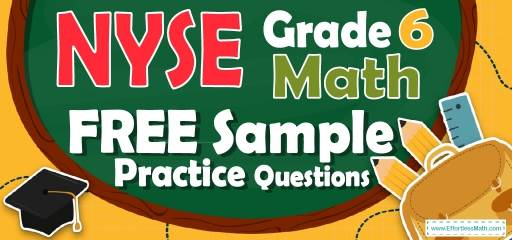# 6th Grade NYSE Math FREE Sample Practice QuestionsStart preparing your student for the 2022 NYSE Math test with our free sample practice questions. Also, make sure to follow some of the related links at the bottom of this post to get a better idea of what kind of mathematics questions students need to practice.

## 10 Sample 6th Grade NYSE Math Practice Questions

1- If the area of the following trapezoid is equal to A, which equation represents $$x$$?
$$\img {https://appmanager.effortlessmath.com/public/images/questions/131313444444444444444444444444444.JPG }$$

A. $$x = \frac{13}{A}$$

B. $$x = \frac{A}{13}$$

C. $$x=A+13$$

D. $$x=A-13$$

2- By what factor did the number below change from first to the fourth number?
$$8, 104, 1352, 17576$$

A. 13

B. 96

C. 1456

D. 17568

3- 170 is equal to …

A. $$-20-(3×10)+(6×40)$$

B. $$((\frac{15}{8})×72 )+ (\frac{125}{5})$$

C. $$((\frac{30}{4} + \frac{15}{2})×8) – \frac{11}{2} + \frac{222}{4}$$

D. $$\frac{481}{6} + \frac{121}{3}+50$$

4- The distance between two cities is 3,768 feet. What is the distance of the two cities in yards?

A. 1,256 yd

B. 11,304 yd

C. 45,216 yd

D. 3,768 yd

5- Mr. Jones saves $3,400 out of his monthly family income of$74,800. What fractional part of his income does Mr. Jones save?

A. $$\frac{1}{22}$$

B. $$\frac{1}{11}$$

C. $$\frac{3}{25}$$

D. $$\frac{2}{15}$$

6- What is the lowest common multiple of 12 and 20?

A. 60

B. 40

C. 20

D. 12

7- Based on the table below, which expression represents any value of f in terms of its corresponding value of $$x$$?
$$\img {https://appmanager.effortlessmath.com/public/images/questions/28282828288888888888888888888888888888888.JPG }$$

A. $$f=2x-\frac{3}{10}$$

B. $$f=x+\frac{3}{10}$$

C. $$f=2x+2 \frac{2}{5}$$

D. $$2x+\frac{3}{10}$$

8- 96 kg = … ?

A. 96 mg

B. 9,600 mg

C. 960,000 mg

D. 96,000,000 mg

9- Calculate the approximate area of the following circle? (the diameter is 25)
$$\img {https://appmanager.effortlessmath.com/public/images/questions/3030303000000000000000000000000000.JPG }$$

A. 78

B. 491

C. 157

D. 1963

10- The following graph shows the mark of six students in mathematics. What is the mean (average) of the marks?
$$\img {https://appmanager.effortlessmath.com/public/images/questions/313131313131111111111111111111111111111111111111.JPG }$$

A. 13

B. 13.5

C. 14

D. 1.5

## Best 6th Grade NYSE Math Exercise Resource for 2022

1- B
The area of the trapezoid is: area$$= \frac{(base 1+base 2)}{2}×$$height$$= (\frac{10 + 16}{2})x = A$$
$$→13x = A→x = \frac{A}{13}$$

2- A
$$\frac{104}{8}=13, \frac{1352}{104}=13, \frac{17576}{1352}=13$$
Therefore, the factor is 13

3- C
Simplify each option provided.
A. $$-20-(3×10)+(6×40)=-20-30+240=190$$
B. $$(\frac{15}{8})×72 + (\frac{125}{5}) =135+25=160$$
C. $$((\frac{30}{4} + \frac{15}{2})×8) – \frac{11}{2} + \frac{222}{4} = ((\frac{30 + 30}{4})×8)- \frac{11}{2}+ \frac{111}{2}=(\frac{60}{4})×8) + \frac{100}{2}= 120 + 50 = 170$$
D. $$\frac{481}{6} + \frac{121}{3}+50= \frac{481+242}{6}+50=120.5+50=170.5$$

4- A
1 yard $$= 3$$ feet
Therefore, $$3,768 ft × \frac{1 \space yd }{3 \space ft}=1,256 \space yd$$

5- A
3,400 out of 74,800 equals to $$\frac{3,400}{74,800}=\frac{17}{374}=\frac{1}{22}$$

6- A
Prime factorizing of $$20=2×2×5$$
Prime factorizing of $$12=2×2×3$$
LCM$$=2×2×3×5=60$$

7- C
Plug in the value of $$x$$ into the function f. First, plug-in 3.1 for$$x$$.
A. $$f=2x-\frac{3}{10}=2(3.1)-\frac{3}{10}=5.9≠8.6$$
B. $$f=x+\frac{3}{10}=3.1+\frac{3}{10}=3.4≠10.8$$
C. $$f=2x+2 \frac{2}{5}=2(3.1)+2 \frac{2}{5}=6.2+2.4=8.6$$
This is correct!
Plug in other values of $$x. (x=4.2)$$
$$f=2x+2\frac{2}{5} =2(4.2)+2.4=10.8$$
This one is also correct.
$$x=5.9$$
$$f=2x+2 \frac{2}{5}=2(5.9)+2.4=14.2$$
This one works too!
D. $$2x+\frac{3}{10}=2(3.1)+\frac{3}{10}=6.5≠8.6$$

8- D
1 kg$$= 1000$$ g and 1 g $$= 1000$$ mg
$$96$$ kg$$= 96 × 1000$$ g $$= 96 × 1000 × 1000 = 96,000,000$$ mg

9- B
The diameter of a circle is twice the radius. Radius of the circle is $$\frac{25}{2}$$.
Area of a circle = $$πr^2=π(\frac{25}{2})^2=156.25π=156.25×3.14=490.625≅491$$

10- B
Average (mean) $$=\frac{sum \space of \space terms}{number \space of \space terms}= \frac{10+11+15+14+15+17+12.5}{7}=13.5$$